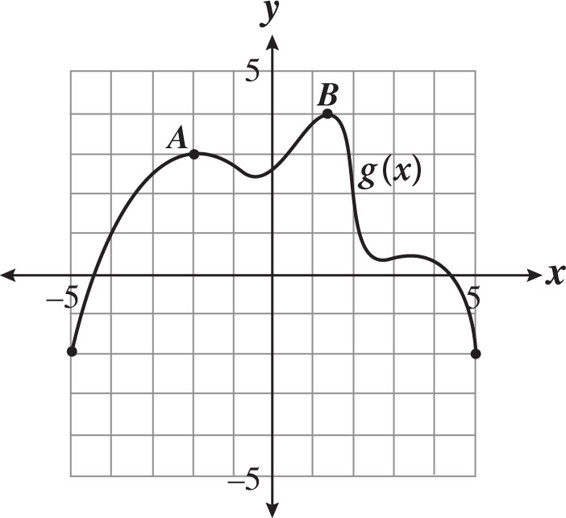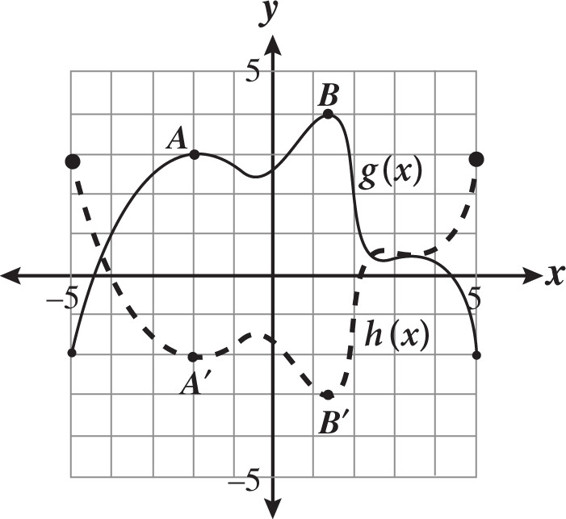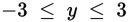# SAT Math Multiple Choice Question 313: Answer and Explanation

### Test Information

Question: 313

13.The graph of g(x) is shown in the figure above. If h(x) = -g(x) + 1, which of the following statements is true?

• A. The range of h(x) is -3 ≤ y ≤ 3.
• B. The minimum value of h(x) is -4.
• C. The coordinates of point A on the function h(x) are (2, 4).
• D. The graph of h(x) is increasing between x = -5 and x = -2.

Explanation:

A

Difficulty: Hard

Category: Passport to Advanced Math / Functions

Strategic Advice: Think about how the transformations affect the graph of g(x) and draw a sketch of h(x) on the same grid. Compare the new graph to each of the answer choices until you find one that is true.

Getting to the Answer: The graph of h(x) = -g(x) + 1 is a vertical reflection of g(x), over the x-axis, that is then shifted up 1 unit. The graph looks like the dashed line in the following graph:Now, compare the dashed line to each of the answer choices: the range of h(x) is the set of y-values from lowest to highest (based on the dashed line). The lowest point occurs at point B' and has a y-value of -3; the highest value occurs at both ends of the graph and is 3, so the range is. This means (A) is correct and you can move on to the next question. Don't waste valuable time checking the other answer choices unless you are not sure about the range. (Choice B: The minimum value of h(x) is -3, not -4. Choice C: The coordinates of point A on h(x) are (-2, -2), not (2, 4). Choice D: the graph of h(x) is decreasing, not increasing, between x = -5 and x = -2.)